• 时间序列分析-------乘法季节模型 实验 名称 乘法季节模型 实验 内容 乘法季节模型 实验 目的 2、熟练建立乘法季节模型 乘法季节...
时间序列分析-------乘法季节模型

实验

名称

乘法季节模型

实验

内容

乘法季节模型

实验

目的

2、熟练建立乘法季节模型

推荐阅读

使用Python完成时间序列分析基础
SPSS建立时间序列乘法季节模型实战案例
SPSS建立时间序列加法季节模型实战案例
Python建立时间序列ARIMA模型实战案例
乘法季节模型结构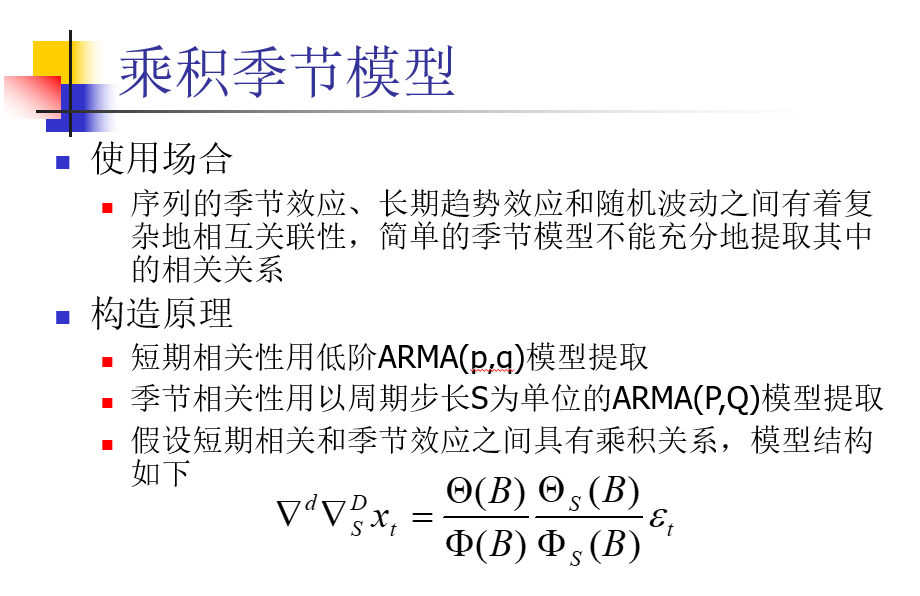乘法季节模型建立

绘制时序图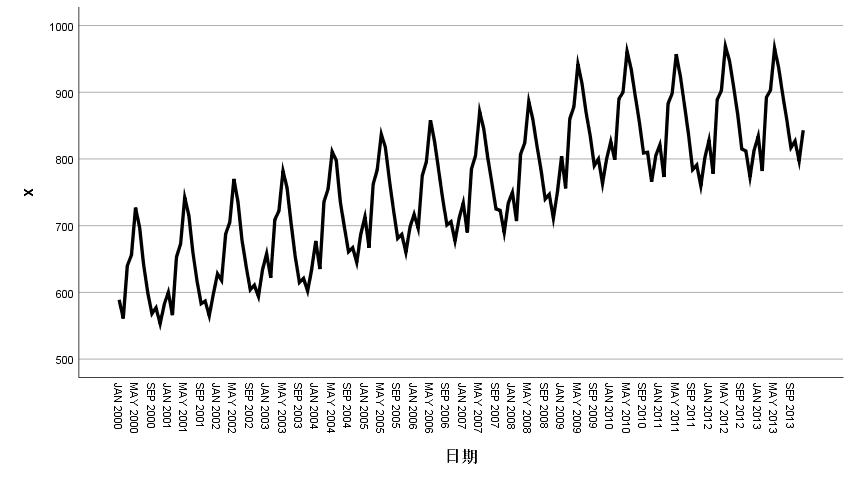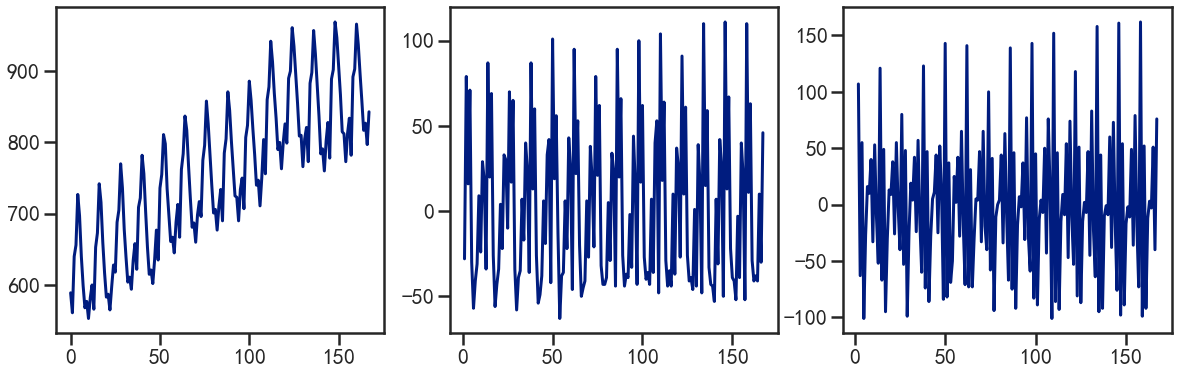时序图显示该序列具有长期增长性趋势和以年为周期的季节效应

差分平稳化

对原序列做1阶12步差分，希望提取原序列的趋势效应和季节效应，差分后的时序图如下所示：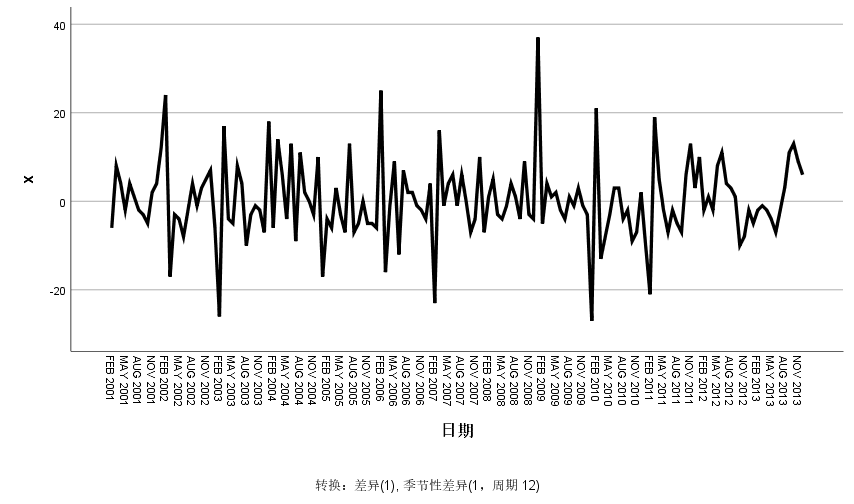易得模型平稳

白噪声检验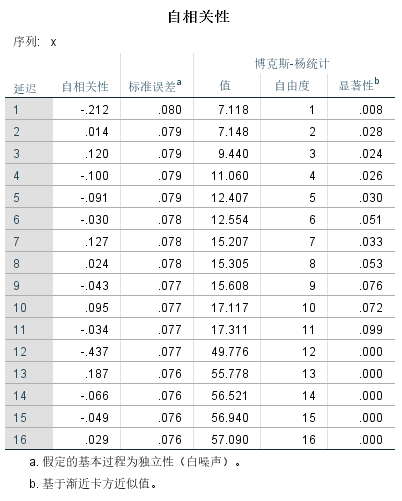P<α，故拒绝原假设，即差分后的序列属于非白噪声序列

综上：差分后的序列是平稳非白噪声序列，需要对差分后的序列进一步拟合ARMA模型

时序图定阶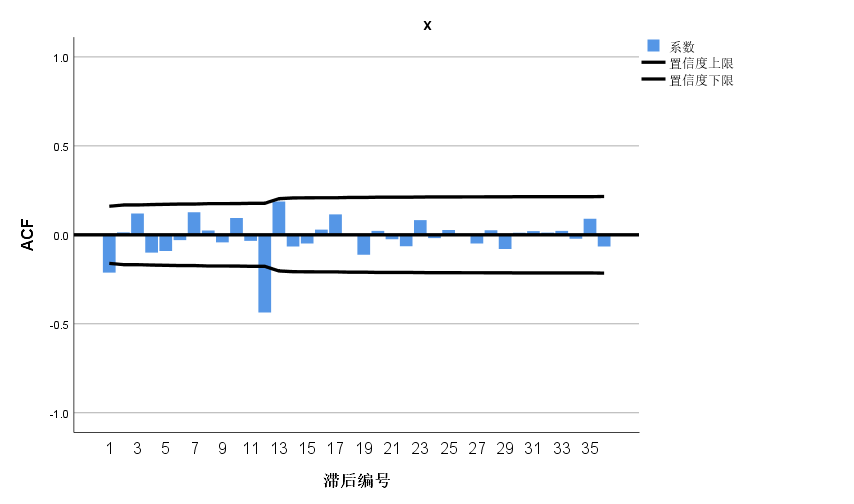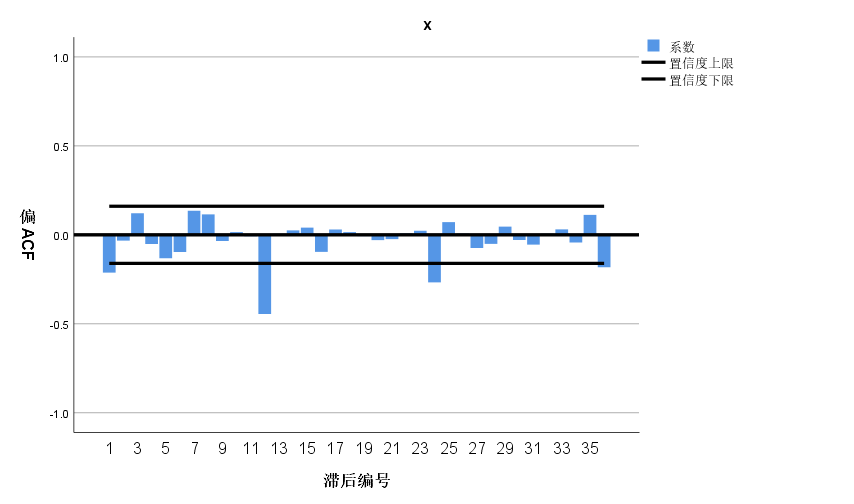自相关图显示延迟12阶自相关系数大于两倍标准差，这说明差分后序列中仍然蕴含非常显著的季节效应，延迟1阶的自相关系数也大于2倍标准差，这说明差分后序列还具有短期相关性。

短期相关特征：自相关图和偏自相关图显示12阶以内的自相关系数，ARMA(1,1),ARMA(1,0),ARMA(0,1)提取差分后序列的短期自相关信息
季节自相关特征：季节自相关特征时自相关系数截尾，偏自相关系数拖尾，这里可以永12步为周期的ARMA(0,1)12模型来提取差分后序列的季节自相关信息。
参数估计和模型检验

综合前面的差分信息，我们要建立ARIMA(1,1,1)*(0,1,1)12、ARIMA(1,1,0)*(0,1,1)12、ARIMA(0,1,1)*(0,1,1)12模型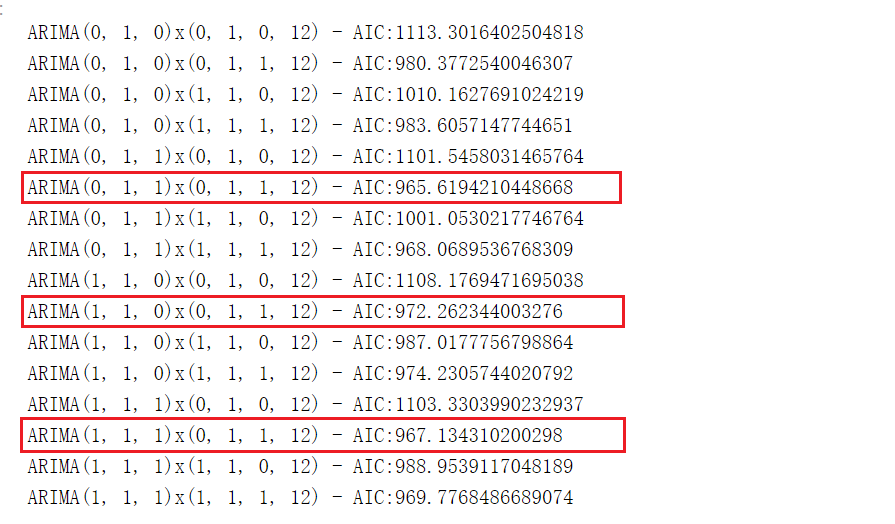ARIMA(1,1,1)*(0,1,1)12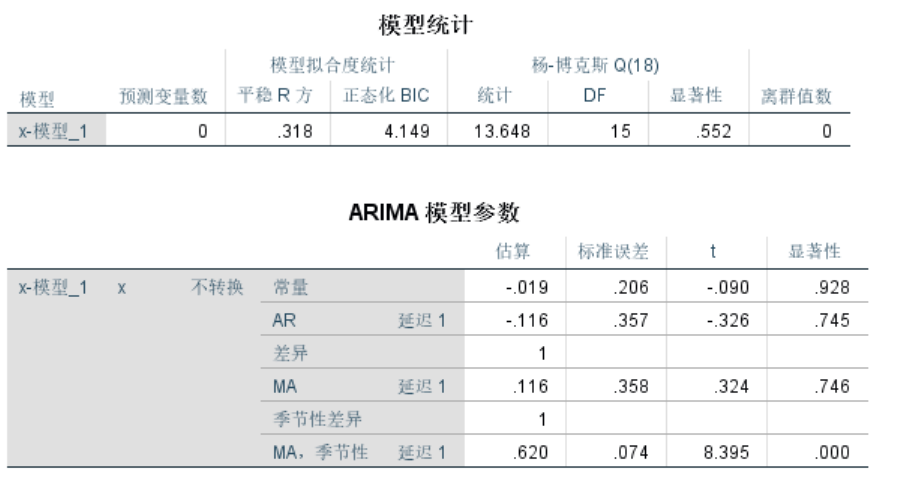P<α，参数的显著性不通过检验

ARIMA(0,1,1)*(0,1,1)12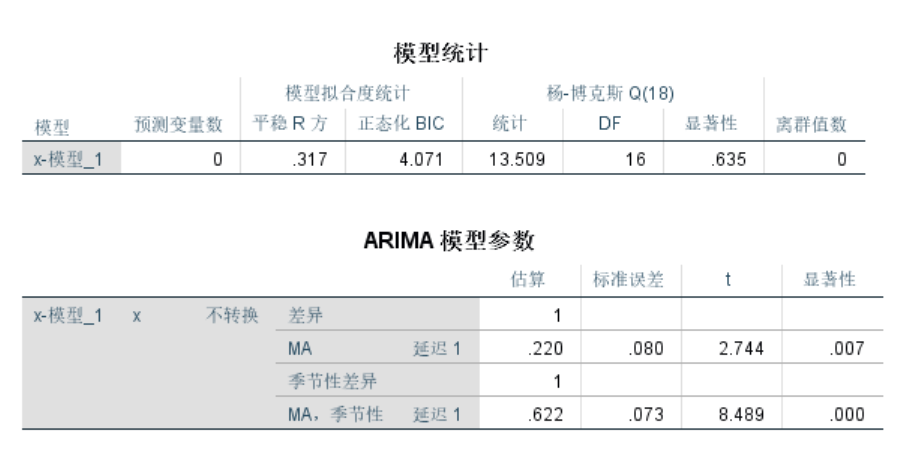模型的显著性检验：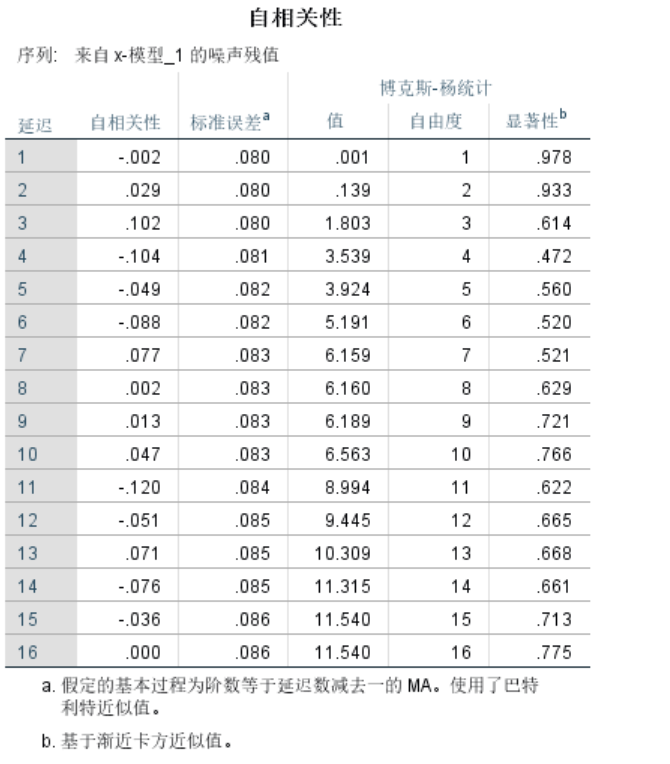建立模型：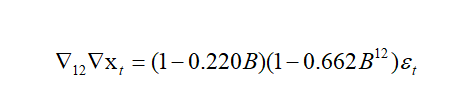ARIMA(1,1,0)*(0,1,1)12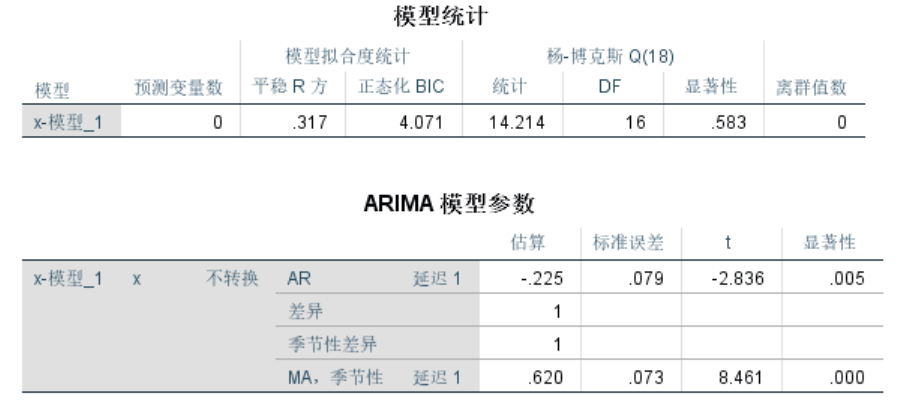参数的显著性检验通过

模型的显著性检验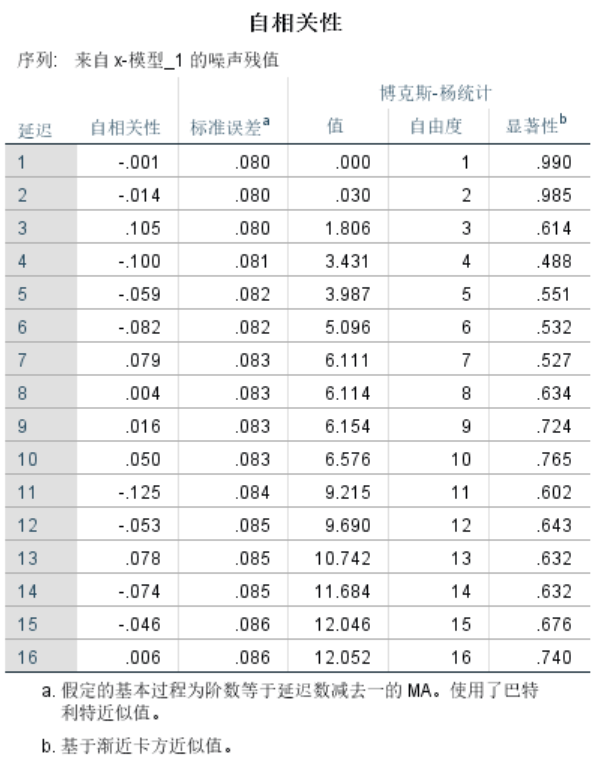建立模型：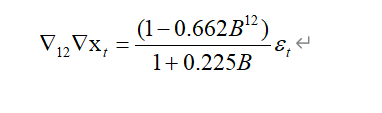模型预测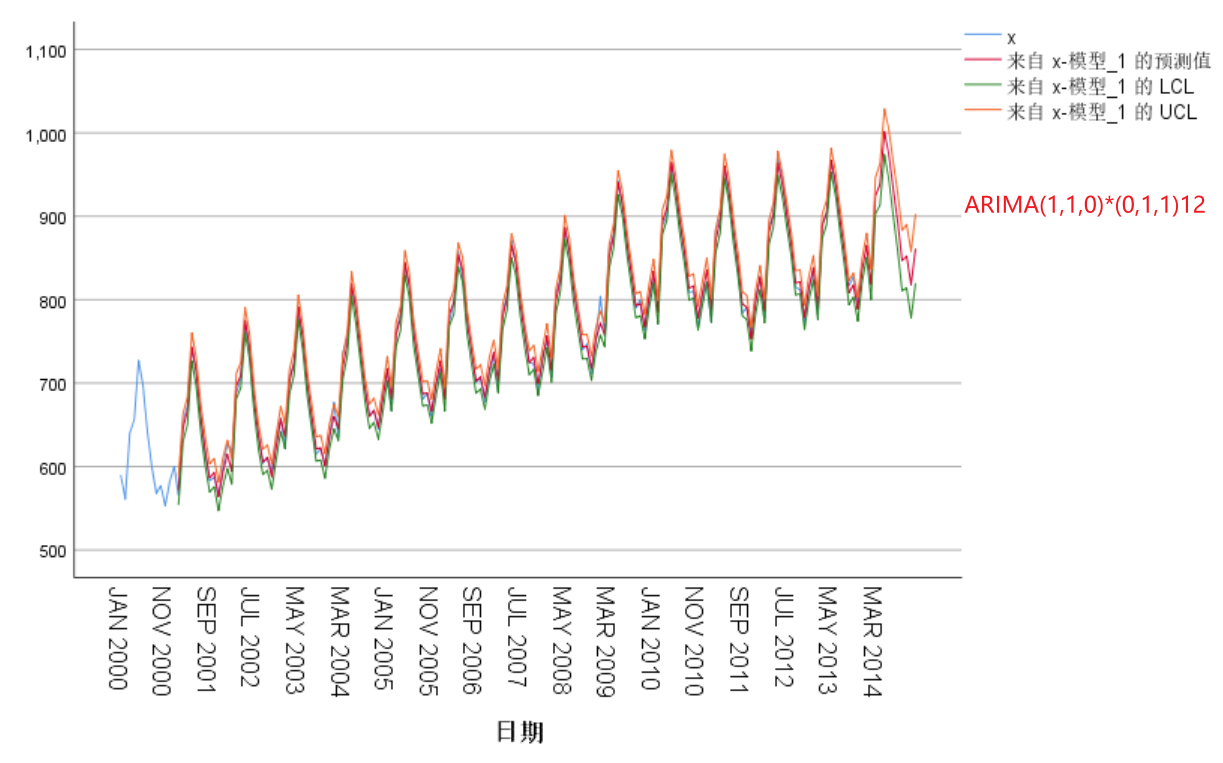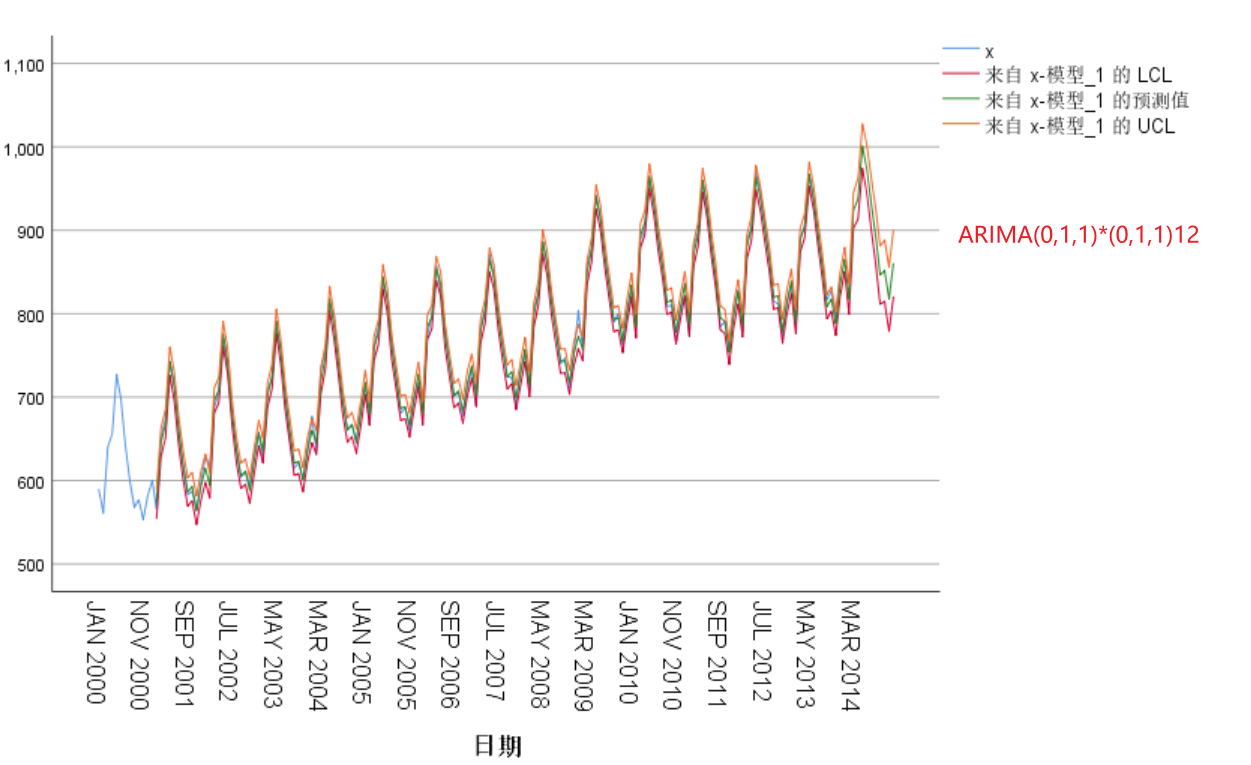> 到这里就结束了，如果对你有帮助你，欢迎点赞关注，你的点赞对我很重要


展开全文数据分析
• 实验 名称 疏系数模型 和季节模型 实验 ... 1、简单季节模型 ... 2、熟练建立季节模型 ...简单季节模型结构 ...使用Python完成时间序列分析基础 SPSS建立时间序列乘法季节模型实战案例 Pyth...

实验

名称

疏系数模型 和季节模型

实验

内容

1、简单季节模型

实验

目的

1、掌握疏系数模型

2、熟练建立季节模型

目录

简单季节模型结构

模型建立

时序图

差分平稳化

白噪声检验

模型定阶

参数估计和模型检验

模型预测

推荐阅读

使用Python完成时间序列分析基础
SPSS建立时间序列乘法季节模型实战案例
Python建立时间序列ARIMA模型实战案例
简单季节模型结构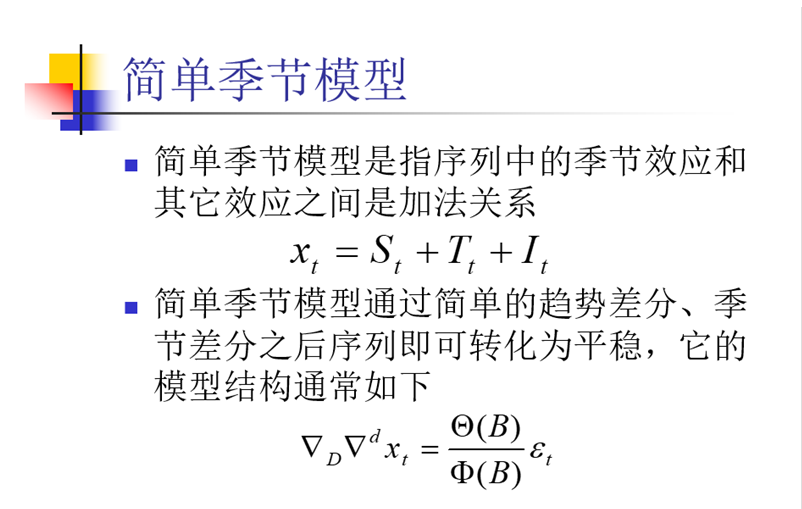模型建立

时序图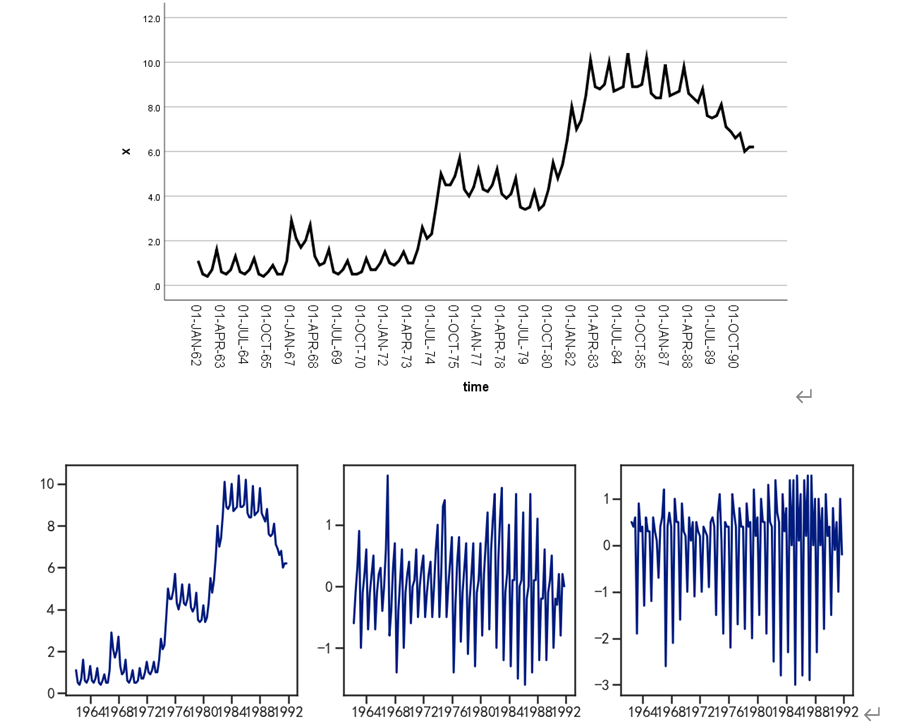时序图显示，该序列既包含长期趋势又包含以年为周期的季节效应

差分平稳化

对原序列做1阶差分消去趋势，再做4步差分消去季节效应的影响，差分后序列时的时序图：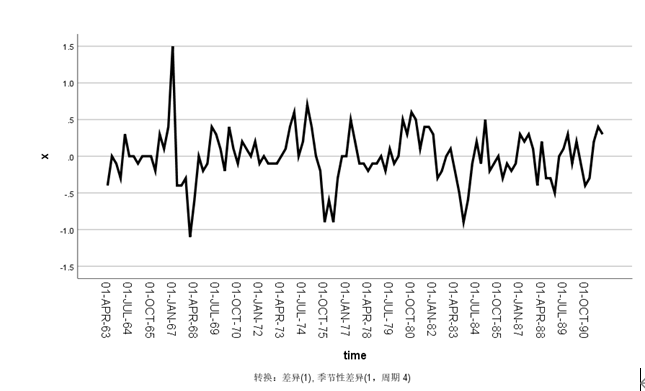单位根检验：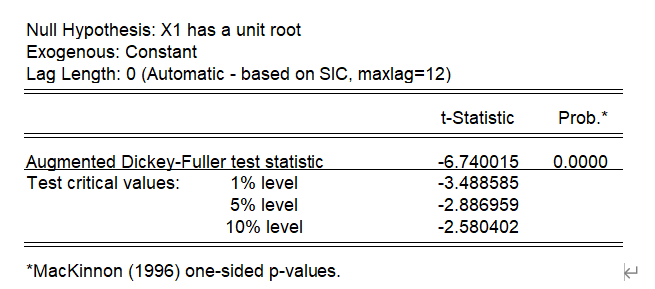白噪声检验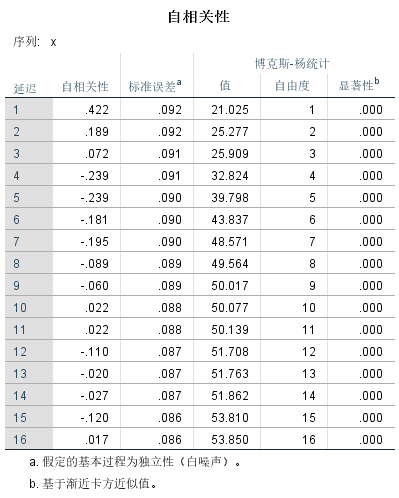检验结果显示，差分后序列时平稳非白噪声序列，需要对差分后的序列进行进一步拟合ARMA模型。

模型定阶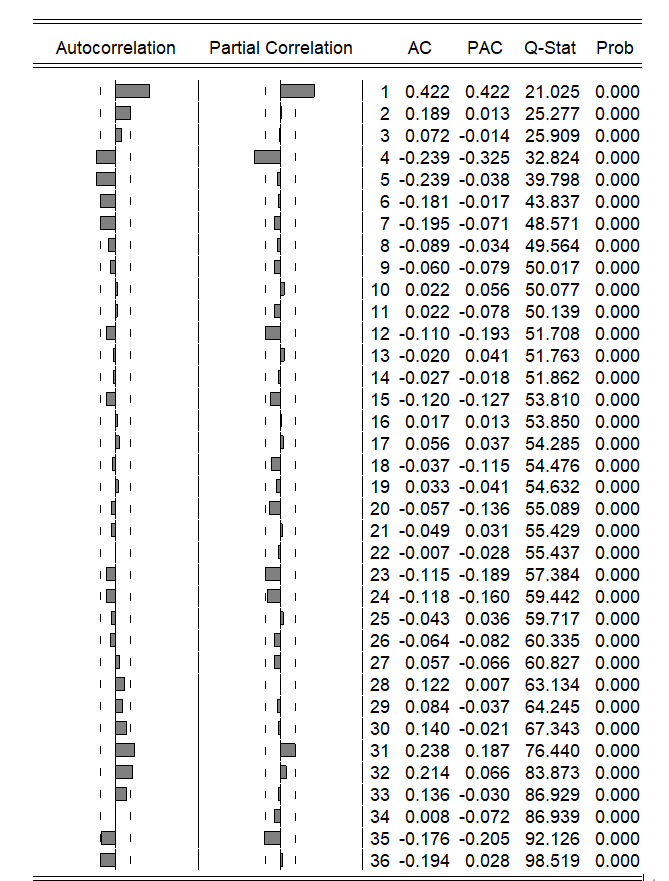自相关图显示出明显的下滑轨迹，这是典型的拖尾属性。偏自相关图除了1阶和4阶偏自相关系数显著大于2倍标准差。所以尝试拟合ARIMA(4,1,0)*(0,1,0)4

参数估计和模型检验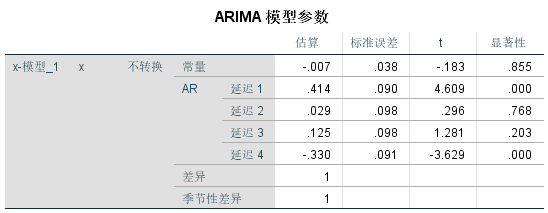x2,x3，P>α，不通过显著性检验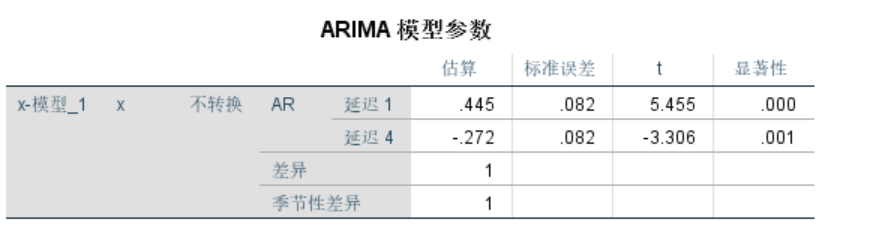模型的显著性检验：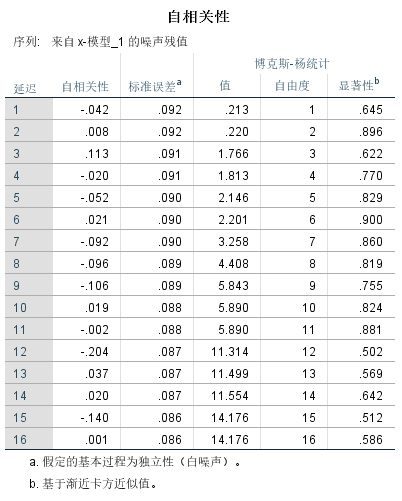检验结果显示，残差序列为白噪声序列，参数显著性检验显示两个参数均显著非0。

模型预测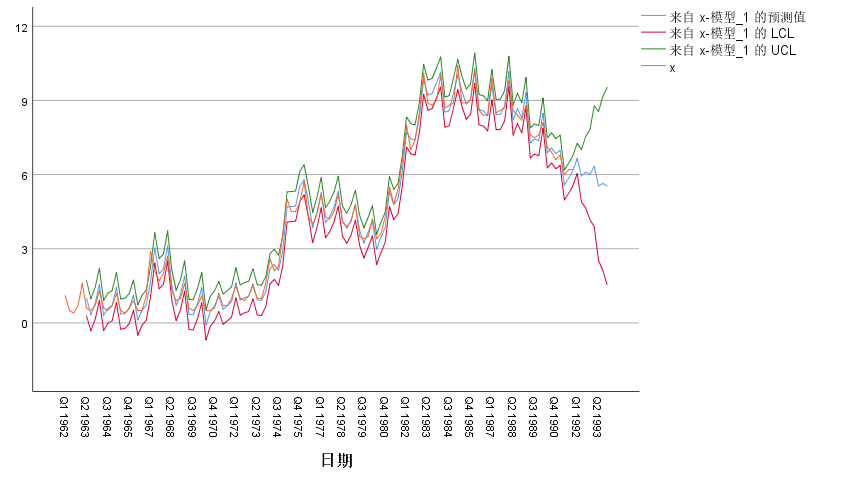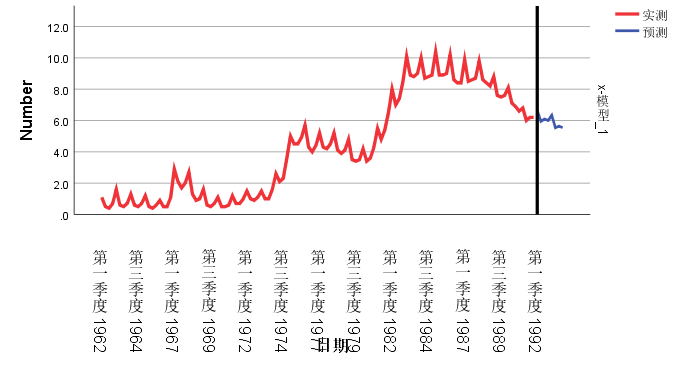> 到这里就结束了，如果对你有帮助你，欢迎点赞关注，你的点赞对我很重要


展开全文• ## 算法模型---时间序列模型

万次阅读 多人点赞 2018-01-16 09:04:58
1、时间序列时间序列是时间间隔不变的情况下收集的不同时间点数据集合，这些...2、随着上升或者下降的趋势，更多的时间序列出现季节性趋势的形式；常用的时间序列模型有AR模型、MA模型、ARMA模型和ARIMA模型等。2、时间
文章来源
时间序列
时间序列是时间间隔不变的情况下收集的不同时间点数据集合，这些集合被分析用来了解长期发展趋势及为了预测未来。
时间序列与常见的回归问题的不同点在于:
1、时间序列是跟时间有关的;而线性回归模型的假设：观察结果是独立的在这种情况下是不成立的。
2、随着上升或者下降的趋势，更多的时间序列出现季节性趋势的形式；
常用的时间序列模型有AR模型(Autoregressive model:自回归模型)、MA模型(moving average model:滑动平均模型)、ARMA模型(Auto-Regressive and Moving Average Model:自回归滑动平均模型)和ARIMA模型(Autoregressive Integrated Moving Average Model:自回归积分滑动平均模型)等。
时间序列的预处理(使数据平稳化和随机化)
拿到一个观察值序列之后，首先要对它的平稳性和纯随机性进行检验，这两个重要的检验称为序列的预处理。根据检验的结果可以将序列分为不同的类型，对不同类型的序列我们会采用不同的分析方法。
平稳化处理
平稳 就是围绕着一个常数上下波动且波动范围有限，即有常数均值和常数方差。如果有明显的趋势或周期性，那它通常不是平稳序列。序列平稳不平稳，一般采用三种方法检验：
时序图检验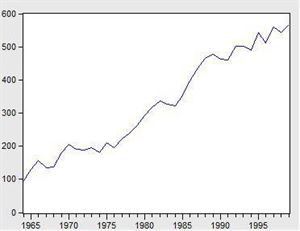看看上面这个图，很明显的增长趋势，不平稳。
利用自相关系数和偏相关系数
自相关系数和偏相关系数的概念可参考《算法模型— 概率论基础—相关系数相关》
下面是不平稳数据的自相关和偏相关系数的一种情形。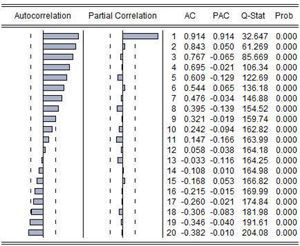左边第一个为自相关图（Autocorrelation），第二个偏相关图(Partial Correlation)。
平稳的序列的自相关图和偏相关图要么拖尾，要么是截尾。截尾就是在某阶之后，系数都为 0 。怎么理解呢，看上面偏相关的图，当阶数为 1 的时候，系数值还是很大， 0.914;二阶长的时候突然就变成了 0.050. 后面的值都很小，认为是趋于 0 ，这种状况就是截尾。什么是拖尾，拖尾就是有一个缓慢衰减的趋势，但是不都为 0 。
自相关图既不是拖尾也不是截尾。以上的图的自相关是一个三角对称的形式，这种趋势是单调趋势的典型图形，说明这个序列不是平稳序列。
平稳序列的自相关系数会快速衰减。
单位根检验
单位根检验是指检验序列中是否存在单位根，如果存在单位根就是非平稳时间序列。
单位根检验：ADF是一种常用的单位根检验方法，他的原假设为序列具有单位根，即非平稳，对于一个平稳的时序数据，就需要在给定的置信水平上显著，拒绝原假设。ADF只是单位根检验的方法之一，如果想采用其他检验方法，可以安装第三方包arch，里面提供了更加全面的单位根检验方法，个人还是比较钟情ADF检验。以下为检验结果，其p值大于0.99，说明并不能拒绝原假设。
利用差分将序列数据转换为平衡序列
差分可以将数据转换为平稳序列。
一阶差分指原序列值相距一期的两个序列值之间的减法运算；k阶差分就是相距k期的两个序列值之间相减。如果一个时间序列经过差分运算后具有平稳性，则该序列为差分平稳序列，可以使用ARIMA模型进行分析。
确定不平稳后，依次进行1阶、2阶、3阶…差分，直到平稳为止。
随机化处理
对于纯随机序列，又称白噪声序列，序列的各项数值之间没有任何相关关系，序列在进行完全无序的随机波动，可以终止对该序列的分析。白噪声序列是没有信息可提取的平稳序列。对于平稳非白噪声序列，它的均值和方差是常数。通常是建立一个线性模型来拟合该序的发展，借此提取该序列的有用信息。ARMA模型是最常用的平稳序列拟合模型。
平稳时间序列建模
某个时间序列经过预处理，被判定为平稳非白噪声序列，就可以进行时间序列建模。
建模步骤：
（1）计算出该序列的自相关系数（ACF）和偏相关系数（PACF）；
（2）模型识别，也称模型定阶。根据系数情况从AR§模型、MA(q)模型、ARMA(p，q)模型、ARIMA（p，d，q）模型中选择合适模型，其中p为自回归项，d为差分阶数，q为移动平均项数。
若平稳序列的偏相关函数是截尾的，而自相关函数是拖尾的，可断定序列适合AR模型；若平稳序列的偏相关函数是拖尾的，而自相关函数是截尾的，则可断定序列适合MA模型；若平稳序列的偏相关函数和自相关函数均是拖尾的，则序列适合ARMA模型。（截尾是指时间序列的自相关函数（ACF）或偏自相关函数（PACF）在某阶后均为0的性质（比如AR的PACF）；拖尾是ACF或PACF并不在某阶后均为0的性质（比如AR的ACF）。）
（3）估计模型中的未知参数的值并对参数进行检验；
（4）模型检验；
（5）模型优化；
（6）模型应用：进行短期预测。
混合自回归移动平均过程（公式表达）
差分方程
所谓差分方程即将变量 $y_t$与它的滞后期联系起来的表达式。
研究变量在第t期的值记为$y_t$。假定给出的动态方程将变量y第t期的值与另外的变量$w_t$以及y的前一期联系起来:
$y_t=ϕy_{t−1}+w_t$
上述称为一阶差分方程是因为仅仅只有变量的一阶滞后$(y_t−1)$出现在方程中。
移动平均
“移动平均”的含义源于$Y_t$是最近两期的$\epsilon$的加权平均。
令${\epsilon_t}$是一个白噪声序列。
$Y_t=μ+\epsilon_t+θ\epsilon_{t−1}$
其中μ和θ可以是任意的常数。这个时间序列称为一阶移动平均过程，记为$MA(1)$。
自回归过程
一阶自回归过程
一个一阶自回归，记作$AR(1)$满足下面的差分方程
$Y_t=c+ϕY_{t−1}+\epsilon_t$
${\epsilon_t}$是一个白噪声序列。
p阶自回归过程
一个p阶自回归，记作$AR(p)$满足下式
$Y_t=c+ϕ_1Y_{t−1}+ϕ_2Y_{t−2}+...+ϕ_pY{t−p}+\epsilon_t$
混合自回归移动平均过程
一个$ARMA(p,q)$过程包括自回归和移动平均项：
$Y_t=c+ϕ_1Y_{t−1}+ϕ_2Y_{t−2}+...+ϕ_pY{t−p}+\epsilon_t+θ_1ϵ_{t−1}+θ_2ϵ{t−2}+...+θ_qϵ_{t−q}$
利用statsmodels库实现时间序列的分析处理
Time Series analysis tsa
statsmodels.tsa.arima_model.ARIMA
AR(I)MA时间序列建模过程——步骤和python代码
Seasonal ARIMA with Python
Python 3中使用ARIMA进行时间序列预测的指南
Python_Statsmodels包_时间序列分析_ARIMA模型
时间序列实战（一）
How to Make Predictions for Time Series Forecasting with Python
How to Use and Remove Trend Information from Time Series Data in Python
时间序列分析之ARIMA上手-Python
[python] 时间序列分析之ARIMA
python中有专门的库statsmodels可以用来作时间序列分析，但spark中则没有现成的。
#-*- coding: utf-8 -*-
import matplotlib.pyplot as plt
import pandas as pd
data=pd.read_csv('./HIS_MONTH.csv')
b=data['fData']
plt.plot(data['fData'])
plt.show()

数据结构如下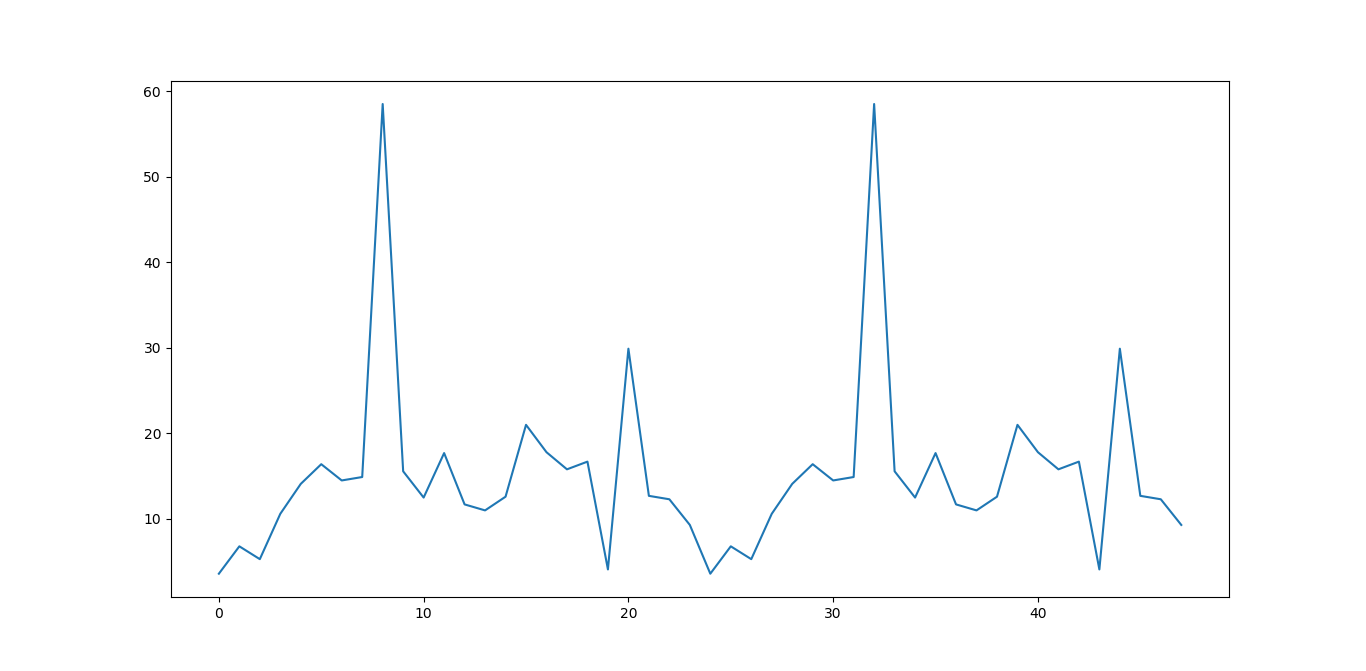#自相关图
from statsmodels.graphics.tsaplots import plot_acf
plot_acf(data['fData']).show()

如下所示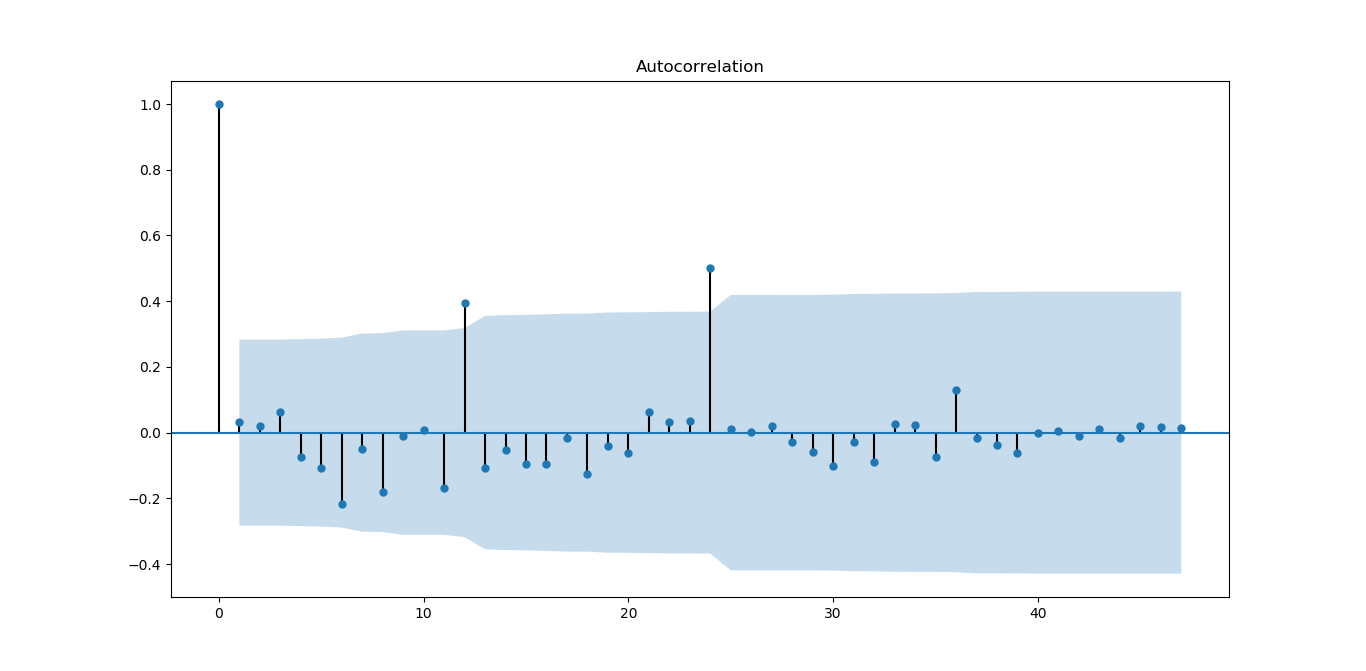从图上可以自相关系数基本是拖尾的，稍做调整就可以使用。
#偏自相关图
from statsmodels.graphics.tsaplots import plot_pacf
plot_pacf(data['fData']).show()

如下图所示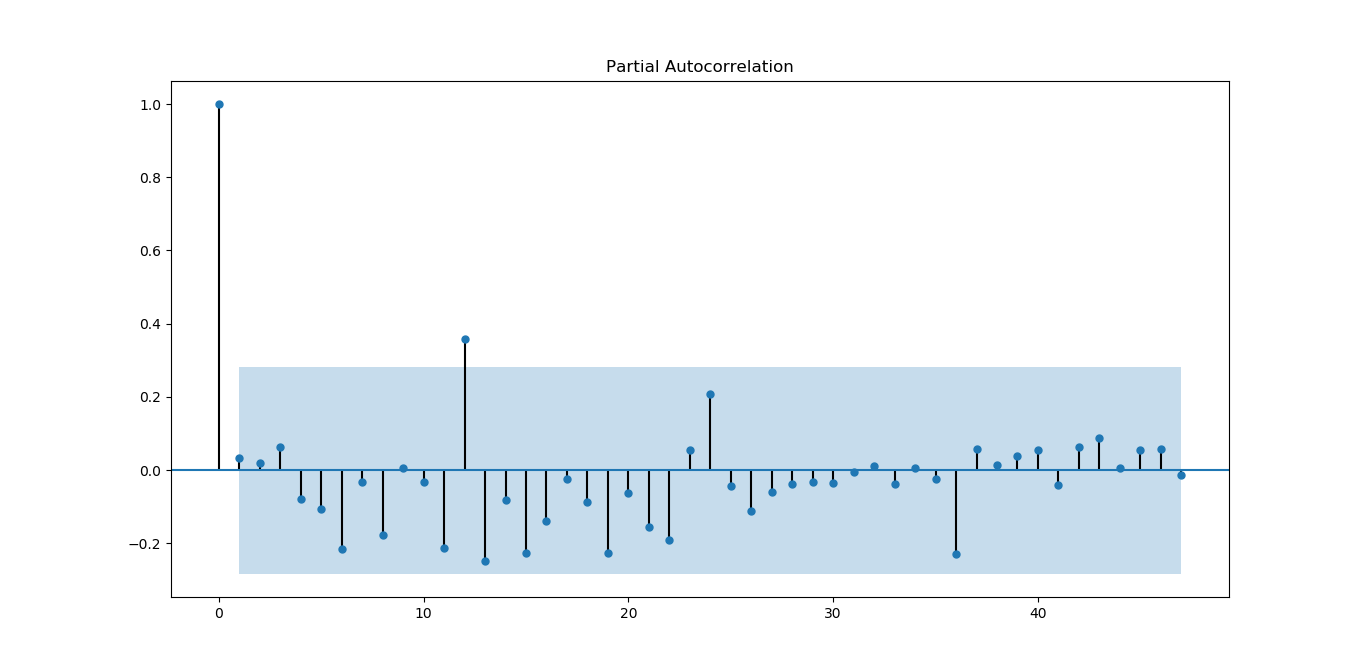偏相关系数也基本符合拖尾。
#平稳性检测
from statsmodels.tsa.stattools import adfuller as ADF
print('原始序列的ADF检验结果为：')
print(ADF(data['fData']))
#返回值依次为adf、pvalue、usedlag、nobs、critical values、icbest、regresults、resstore

如下所示
原始序列的ADF检验结果为：
(-6.561077625309946, 8.378411469638636e-09, 0L, 47L, {'5%': -2.925338105429433, '1%': -3.5778480370438146, '10%': -2.6007735310095064}, 273.5266547319719)

p值远小于0.05，拒绝原假设（原假设认为存在单位根），所以该序列为平衡时间序列。
#白噪声检验
from statsmodels.stats.diagnostic import acorr_ljungbox
print('差分序列的白噪声检验结果为：')
print(acorr_ljungbox(data['fValueData'], lags=1))

结果如下
差分序列的白噪声检验结果为：
(array([0.05198092]), array([0.81965149]))

P=0.81965149，统计量的P值大于显著性水平0.05，则接受原假设（原假设认为为白噪声序列）。
下面对原序列做一阶差分运算
#差分后的结果
D_data = data['fValueData'].diff().dropna()
D_data.columns = ['fValueData']
#时序图
D_data.plot()

结果如下：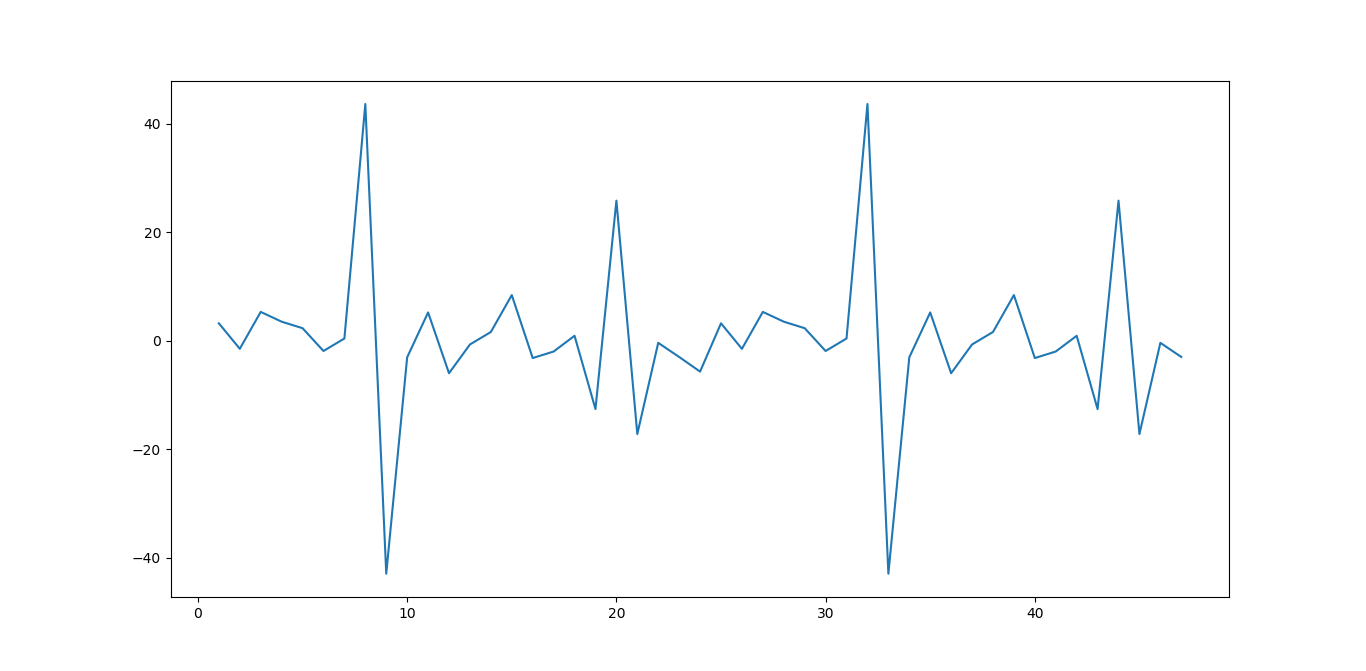从图上看，一阶差分后，感觉数据分布更对称为随机了。
#自相关图
plot_acf(D_data).show()
plt.show()

结果如下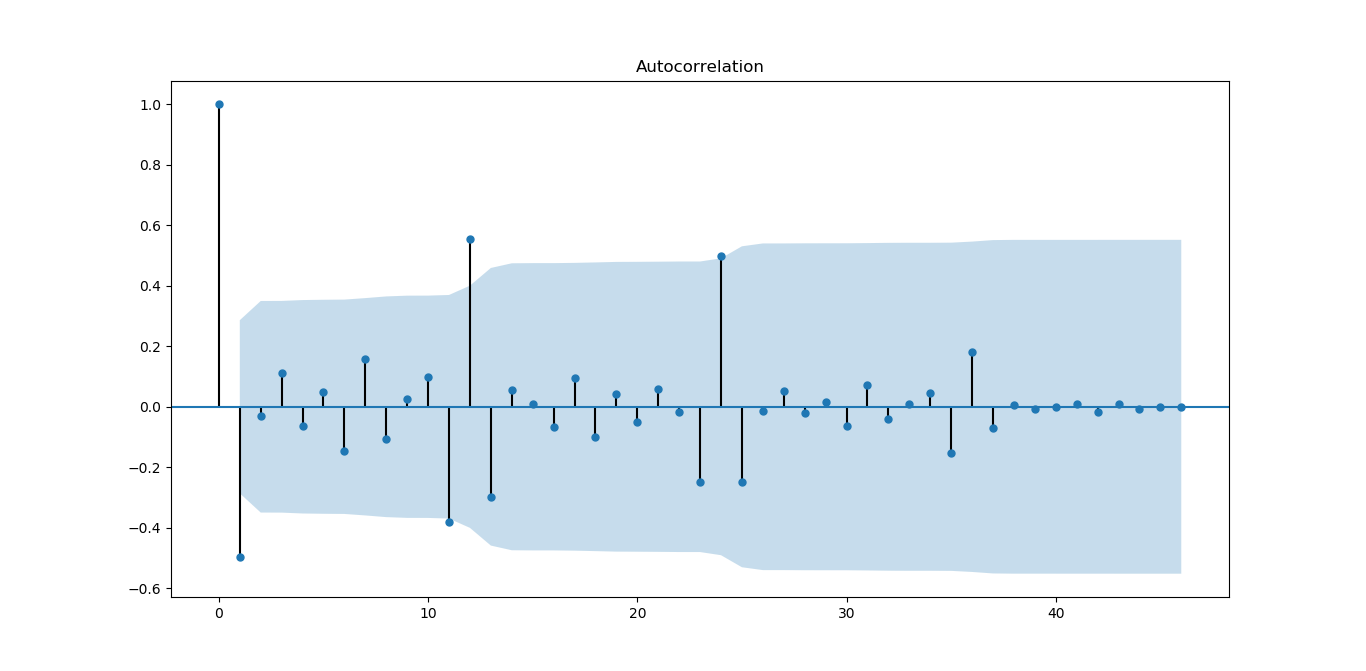从图上看，一阶差分对数据有改善，振荡衰减更明显
#偏自相关图
plot_pacf(D_data).show()

结果如下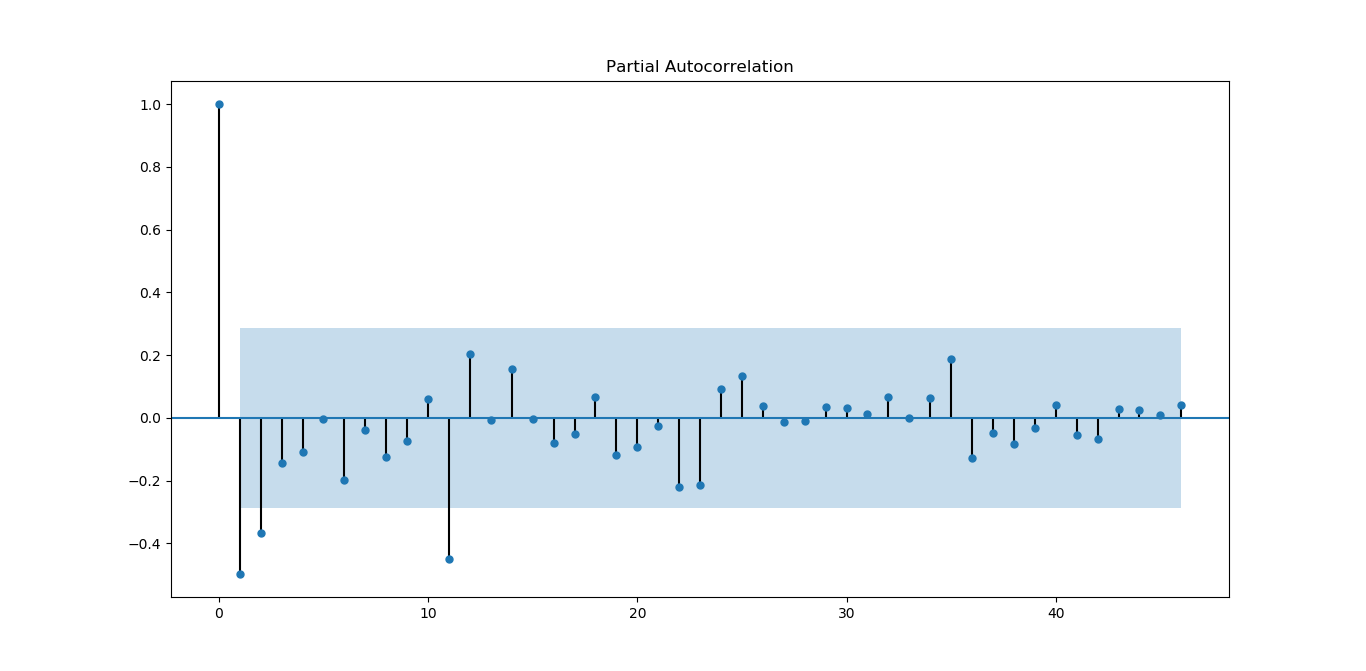从图上看，偏相关系数虽然没有自相关系数好，但基本能满足平稳序列的要求。
#平稳性检测
print('差分序列的ADF检验结果为：')
print( ADF(D_data))

结果如下
差分序列的ADF检验结果为：
(-4.595500765524432, 0.0001316587309452837, 10L, 36L, {'5%': -2.9459512825788754, '1%': -3.626651907578875, '10%': -2.6116707716049383}, 270.35268975914374)

p值远小于0.05，拒绝原假设（原假设认为存在单位根），所以该序列为平衡时间序列。
#白噪声检验
from statsmodels.stats.diagnostic import acorr_ljungbox
#返回统计量和p值
print('差分序列的白噪声检验结果为：')
print(acorr_ljungbox(D_data, lags=1))

结果如下：
差分序列的白噪声检验结果为：
(array([12.35619393]), array([0.00043953]))

P=0.00043953，统计量的P值小于显著性水平0.05，则拒绝原假设（原假设认为为白噪声序列），所以一阶差分后该序列为非白噪声序列，有进一步分析的价值。
Holt-Winters模型原理及python实现
本部分来源，也可以参考该文
原理分析
公式前面也写过，这里再表述一下，有时间再来整理
假设随机时间序列为：
$x_t(t=1,2,\ldots,N,N=n)$
时间序列数据一般有以下几种特点：1.趋势(Trend)  2. 季节性(Seasonality)。
趋势描述的是时间序列的整体走势，比如总体上升或者总体下降。下图所示的时间序列是总体上升的：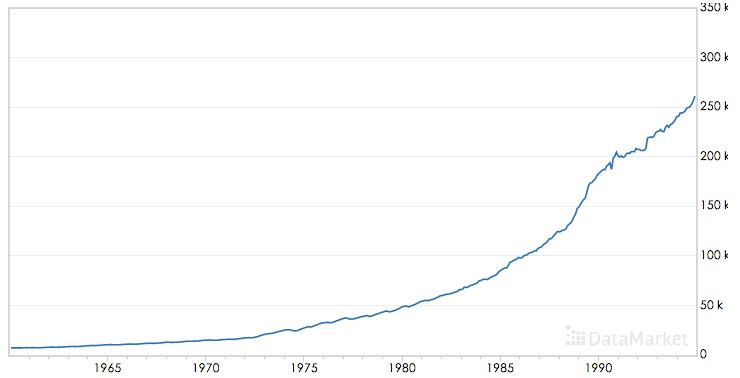季节性描述的是数据的周期性波动，比如以年或者周为周期，如下图：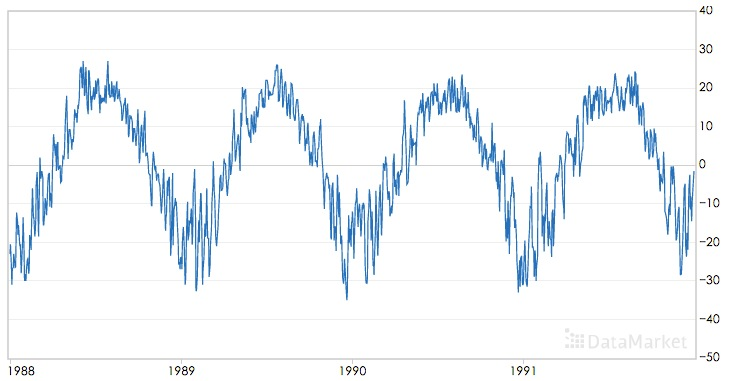三次指数平滑算法可以对同时含有趋势和季节性的时间序列进行预测，该算法是基于一次指数平滑和二次指数平滑算法的。
移动平均(The simple moving average (MA))
直观上,最简单的平滑时间序列的方法是实现一个无权重的移动平均,目前已知的方法是用窗口函数,平滑统计量 $S_t$就是最近k个观察值的均值。公式如下:
$s_t=\frac{1}{k} \sum_{n=0}^{k-1} x_{t-n}=\frac{x_t+x_{t-1}+\ldots + x_{t-k+1}}{k}=s_{t-1}+\frac{x_t -x_{t-k}}{k}$
这样的方法存在明显的缺陷,当k比较小时,预测的数据平滑效果不明显,而且突出反映了数据最近的变化;当k较大时,虽然有较好的平滑效果,但是预测的数据存在延迟。而且最少需要k个值(窗口有限)。
加权移动平均
一种稍微复杂的方法是先选择一组权重因子来计算加权移动平均
${\omega_1,\omega_2,\ldots,\omega_k},满足\sum_{n=1}^{k}\omega_n=1$
然后用这些权重来计算这些平滑统计量:
$s_t=\sum_{n=1}^{k}\omega_n x_{t-n+1}=\omega_1x_t+\omega_2 x_{t-1}+\ldots+\omega_{k}x_{t-k+1}$
在实践中,通常在选择权重因子时,赋予时间序列中的最新数据更大的权重,并减少对旧数据的权重。这个方法也需要最少k个值,并且计算复杂。
简单指数平滑法
幸运地是有一种方法可以避免上述问题,它叫做指数平滑法。最简单的指数平滑法如下:
$s_i = \alpha x_i + (1-\alpha)s_{i-1}$
其中$\alpha$是平滑因子,$0 < \alpha < 1$。$s_i$是当前时刻的平滑值。换句话说,平滑统计值$S_i$是当前统计值$X_t$与上一时间平滑值$S_{i-1}$加权平均。这个简单指数平滑是很容易被应用的,因为只要有两个观察值就能计算了。这里α的选取,我们可以采用最小二乘来决定$\alpha$(最小化$(s_t-x_t)^2$)。
一次指数平滑算法进行预测的公式为：
$x_{i+h}=s_i$
其中i为当前最后的一个数据记录的坐标，亦即预测的时间序列为一条直线，不能反映时间序列的趋势和季节性。
为什么被称为“指数”平滑法
从它的递推公式就能发现:
简单指数平滑法适用于没有总体趋势的时间序列。如果用来处理有总体趋势的序列,平滑值将往往滞后于原始数据,除非α的值接近1,但这样一来就会造成不够平滑。
二次指数平滑（HoltWinters-无季节趋势）
为了解决上述问题,于是引出了二次指数平滑,能够保留总体趋势信息。因为将指数平滑应用了两次,所以被称为二次指数平滑。与简单指数平滑相比,二次指数平滑加入了时间趋势统计量$t_i$,公式如下:
$s_i=\alpha x_i + (1-\alpha)(s_{i-1}+t_{i-1})\\ t_i=\beta (s_i-s_{i-1}) + (1-\beta)t_{i-1}$
$s_i$添加了与一次指数平滑相比，添加了一个新的趋势项$t_i$；而$t_i$的表达式与一次指数平滑形式上是一样的，只不过用将$x_i$换成$(s_i-s_{i-1})$,趋势可以想像成增量，就是在此刻与上一刻的差值，趋势当然应该用平滑后的数据的差值；同时将一次平滑中的$s_{i-1}$换成$t_{i-1}$
二次指数平滑的预测公式为
$x_{i+h}=s_i+h \ t_i$
二次指数平滑的预测结果是一条斜的直线
三次指数平滑
三次指数平滑将时间序列的季节性这一特征也考虑进去了。
季节性被定义为时间序列数据的趋势,它表现出每一个周期重复自身的行为,就像任何周期函数一样。“季节”这个词用来表示行为每隔时间段L就开始自我重复。在自然界中有不同类型的季节性“累加性”(additive)和“累乘性“(multiplicative),就像加法和乘法是数学的基本运算。
如果每个12月都比每个11月多卖出1000套公寓,我们就说这样的季节趋势是“累加性”的。可以用绝对增⻓来表示。如果我们在夏季比冬季多卖出10%的公寓,那么季节趋势在自然中是“累乘性”的。
累乘性公式如下:
下面的式子中$k$表示季节频率，这个还需要查资料进行理解
$s_i=\alpha \frac{x_i }{p_{i-k}} +(1-\alpha)(s_{i-1}+t_{i-1})\\ t_i=\beta (s_i-s_{i-1}) + (1-\beta)t_{i-1}\\ p_i=\gamma \frac{x_i}{s_i} + (1-\gamma)p_{t-k}\\$
上式中k为周期， 累乘三次指数平滑的预测公式为：
$x_{i+h}=(s_i+h\ t_i)p_i-k+(h\ mod\ k)$
或者
$x_{i+h}=(s_i+h\ t_i)p_{i-k+1+(h-1) \ mod \ k}$
两个公式还需要确认下
累乘性公式初始值的计算
$s_k=\frac{1}{k} (s_1+s_2+\cdots+s_k)\\ t_k=\frac{1}{k}\left[ \frac{x_{k+1}-x_1}{k}+\frac{x_{k+2}-x_2}{k}+\cdots+\frac{x_{k+k}-x_k}{k} \right]\\ p_1=\frac{x_1}{s_k},p_2=\frac{x_2}{s_k},\cdots,p_k=\frac{x_k}{s_k}$
累加性公式如下:
$s_i=\alpha (x_i-p_{i-k} )+(1-\alpha)(s_{i-1}+t_{i-1})\\ t_i=\beta (s_i-s_{i-1}) + (1-\beta)t_{i-1}\\ p_i=\gamma (x_i-s_i) + (1-\gamma)p_{i-k}\\ x_{i+h}=s_i+hb_i + p_{i-k+h \ mod \ k}$
或者
$x_{i+h}=s_i+hb_i + p_{i-k+1+(h-1) \ mod \ k}$
两个公式还需要确认下
累加性公式初始值的计算
$s_k=\frac{1}{k} (s_1+s_2+\cdots+s_k)\\ t_k=\frac{1}{k}\left[ \frac{x_{k+1}-x_1}{k}+\frac{x_{k+2}-x_2}{k}+\cdots+\frac{x_{k+k}-x_k}{k} \right]\\ p_1=x_1-s_k,p_2=x_2-s_k,\cdots,p_k=x_k-s_k$
其中 $\alpha$是数据平滑因子, $0 < \alpha < 1$;$\beta$是趋势平滑因子,$0 < \beta < 1$; $\gamma$是季节改变平滑因子$0 < \gamma < 1$。
$\alpha，\beta，\gamma$的值都位于[0,1]之间，可以多试验几次以达到最佳效果。
$s,t,p$初始值的选取对于算法整体的影响不是特别大，通常的取值为$s_0=x_0,t_0=x_1-x_0$,累加时$p=0$,累乘时$p=1$.
对三次指数平滑法而言,我们必须初始化一个完整的“季节”$p_i$的值,不过我们可以简单地设置为全1(针对累乘式)或全0(针对累加式)。只有当序列的⻓度较短时,我们才需要慎重考虑初始值的选取。
我们这里讲的Holt-Winters模型就是三次指数平滑法。哇,终于切入正题了。
所有的指数平滑法都要更新上一时间步⻓的计算结果,并使用当前时间步⻓的数据中包含的新信息。它们通过“混合”新信息和旧信息来实现,而相关的新旧信息的权重由一个可调整的拌和参数来控制。各种方法的不同之处在于它们跟踪的量的个数和对应的拌和参数的个数。三次指数平滑法,功能最强大,既能体现趋势性又能体现季节性,所以三次指数平滑法的参数最多,有三个。
下图为使用累加三次指数平滑进行预测的效果：其中红色为源时间序列，蓝色为预测的时间序列，$\alpha，\beta，\gamma$的取值为0.45，0.2，0.95：下图为累乘三次指数平滑进行预测的效果，$\alpha，\beta，\gamma$的取值为0.4，0.05，0.9：可以看到三次指数平滑算法可以很好的保存时间序列数据的趋势和季节性信息，在International Airline Passengers数据集上累乘平滑指数算法的效果更好。
python代码实现
我们知道HoltWinters模型有三个可调参数,我们的目的就是训练出有效的α,β, γ
。我们有两种方法,一种就是自己取值来试试,一种就是采用数值优化的思想,比如
前面我们提到的最小二乘来最小化误差来求参数(注意不一定能全局收敛!这个问题
实在是让人头痛。。。)我们就采用最小二乘法(L-BFGS)。
RMSE的实现
statsmodels中的holtwinters
statsmodels.tsa模块
statsmodels.tsa.holtwinters源码
statsmodels.tsa.holtwinters.ExponentialSmoothing
ExponentialSmoothing - 指数平滑算法
基于SVM作短期时间序列的预测
传统的做法是提取1、2、3、4、5、7、9、13个单位时间的数据作为特征进行预测；
举个例子进行分析，比如每天都有口香糖的销量，那么如何通过几周的数据预测明天的数据，
就可以选择前1、2、3、4、5、7、14天的数据作为特征，从而预测明天的数据，
通过构建特征，再选择核函数进行预测，其中调参的参数尽量要进行最优化，
参考方法：如果选择RBF核函数，那么其中就会有三个参数，固定两个，然后不停的优化另外一个，直到得到最优解。
具体应用的例子：
（1）SVM预测风场：http://wenku.baidu.com/link?url=SCCIJJe8tXLbTjLMZ81x5Qy6elsceAKIOwtkZ0QxfSCQQ4KaWKwo8Biepjs3Ss2LJ2ewhisNR0ixrDY4kV1Rd7BcqWRenuTaG85K80E-30y
（2）SVM预测股票指数：基于SVM修正的模糊时间序列模型在沪指预测中的应用
（3）SVM预测时间序列其他方面：http://www.docin.com/p-233353900.html
LSTM模型分析及对时序数据预测的具体实现（python实现）
来源：2017年09月30日 10:28:08
如何用LSTM自编码器进行极端事件预测？(含Python代码)
时间序列的并行实现
spark里面的库是没有时间序列算法的，但是国外有人已经写好了相应的算法。其github网址是:https://github.com/sryza/spark-timeseries
sryza/spark-timeseries
Spark-TimeSeries使用方法
A New Library for Analyzing Time-Series Data with Apache Spark
【Spark Summit East 2017】使用Spark进行时间序列分析
基于小波变换的时间序列预测，Python实现，来自雪球
清华AIOps算法：KPI聚类
其他资料
时间序列挖掘-预测算法-三次指数平滑法(Holt-Winters)
Holt-Winters原理和初始值的确定
时间序列模型Prophet使用详细讲解


展开全文• 时间序列预测模型 1.时间序列分解 2.ARIMA模型 时间序列（或称动态数列）是指将同一统计指标的数值按其发生的时间先后顺序排列而成的数列。时间序列分析的主要目的是根据已有的历史数据对未来进行预测。 一个时间...
时间序列预测模型
1.时间序列分解
2.ARIMA模型
时间序列（或称动态数列）是指将同一统计指标的数值按其发生的时间先后顺序排列而成的数列。时间序列分析的主要目的是根据已有的历史数据对未来进行预测。
一个时间序列往往是以下几类变化形式的叠加或耦合：

长期趋势(Secular trend,T):长期趋势指现象在较长时期内持续发展变化的一种趋向或状态；
季节变动(Seasonal Variation,S):季节变动是由于季节的变化引起的现象发展水平的规则变动；
循环波动(Cyclical Variation,C):循环波动是指以若干年为期限，不具严格规则的周期性连续波动；
不规则波动(Irregular Variation,I):不规则波动也称随机波动，是指由于众多偶然因素对时间序列造成的影响。

1.时间序列分解模型
可采用加法结构或乘法结构分解时间序列：

加法模型的形式如下：
$x_t=T_t+C_t+S_t+I_t$
加法模型中的四种成分之间是相互独立的，某种成分的变动并不影响其他成分的变动。其中,对于等式右侧，第一项是趋势项，第二项是周期项，第三项是季节项，第四项是随机项。

乘法模型的形式如下：
$x_t=T_t*S_t*C_t*I_t$
乘法模型中四种成分之间保持着相互依存的关系。
此外，还有加乘混合模型：

加乘混合模型的形式如下：
$x_t=T_t*C_t*S_t+I_t$
$x_t=S_t+T_t*C_t*I_t$

STL(Seasonal and Trend decomposition using Loess)分解：
最常用的分解方法；
将时间序列分解为周期项（季节项）+趋势项+随机项

STL分解可将时间序列分为三类：

没有趋势、也没有周期的序列（水平型时间序列）
只有趋势、没有周期的序列（斜坡型时间序列）
既有趋势，又有周期的序列（含趋势和周期的）

可以使用指数平滑预测各项：

水平型时间序列：简单指数平滑
斜坡型时间序列：Holt两参数指数平滑
含趋势和周期的时间序列：Holt-winters三参数指数平滑

ARIMA模型
Auto Regressive Intergrated Moving Average Model（自回归差分移动平均模型）
ARIMA模型是在平稳的时间序列基础上建立的，因此时间序列的平稳性是建模的前提。检验时间序列模型平稳的方法一般采用ADF单位根检验模型去检验。此外，如果时间序列不稳定，可以通过一些操作使得时间序列稳定（如常用的取对数、差分处理），然后进行ARIMA模型预测，得到稳定的时间序列的预测结果，然后对预测结果进行之前使序列平稳的逆操作（取指数、差分的逆操作），即可得到原始数据的预测结果。
ARIMA模型的预处理流程如下图所示：观察时间序列数据是否平稳的方式：

时序图
自相关系数图（平稳序列通常短期相关）
单位根检验：
1.检验序列是否平稳
2.原假设：给定时间序列是非平稳序列
3.ADF检验
4.KPSS检验
5.若不平稳，则可尝试差分运算

差分运算：

相距1期之间两个序列值之间的减法运算称为一阶差分运算
$\nabla x_t=x_t-x_t-1$

对一阶差分序列再进行一次一阶差分运算称为二阶差分运算
$\nabla^2x_t=\nabla x_t-\nabla x_t-1$

相距k期之间两个序列值之间的减法运算称为k步差分运算
$\nabla_kx_t=x_t-x_t-k$

自相关系数
时间序列观测值与其过去的观测值之间的线性相关性
偏自相关系数
在去除中间k-1个随机变量的干扰后,t-k时刻观测值与t时刻观测值间的相关性


展开全文数据分析
• 时间序列定义： 时间序列是按照一定的时间间隔排列的一组数据，其时间间隔可以是任意的时间单位，如小时、日、周月等。比如，每天某产品的用户数量，每个月的销售额，这些数据形成了以一定时间间隔的数据。 通过对...python 数据分析
• 时间序列可以分为长期趋势（trend）、季节变动（seasonal）、循环变动（cycling）和随机波动（irregular）四个部分。 长期趋势（ T ）：在较长时期内受某种根本性因素作用而形成的总的变动趋势 季节变动（ S ）：在...数据分析 机器学习
• 测试序列稳定性：看以看到整体的序列并没有到达稳定性要求，要将时间序列转为平稳序列，有如下几种方法：DeflationbyCPILogarithmic（取对数）FirstDifference（一阶差分）SeasonalDifference（季节差分）...
• 本文聊聊arima模型。ARIMA是一种比较常用的基于历史数据来做预测的时间序列模型时间序列在商业中有很多应用，比如它可以解释销售中的季节性规律；可以预测新客或流失客户...
• ## R语言 时间序列arima模型

万次阅读 多人点赞 2018-06-20 20:55:27
基本理论知识   ARMA模型称为自回归移动平均模型，是时间序列里常用的模型之一。ARMA模型是对不含季节变动的平稳序列进行建模。它将序列值表示为过去值和过去扰动项的加权和。模型形式如下：yt=c+a1yt−1+a2yt−2...R语言
• 实验 名称 疏系数模型 和季节模型 ... SPSS建立时间序列乘法季节模型实战案例 Python建立时间序列ARIMA模型实战案例 疏系数模型的定义 时序图 白噪声检验 ...机器学习
• 论文研究-结构时间序列模型季节调整方面的应用——与X-12季节调整方法的比较分析 .pdf,
• 1. TBATS简介 名字来源: Trigonometric seasonality, Box-...模型使用季节性特征、Box-Cox转换、ARMA误差、趋势和季节组分。 2. 模型 可以看出预测的 y 由4部分组成， l 是局部水平， b 是趋势水平， d 是ARMA模型...
• 不同时间序列预测模型的示例。 前处理 时间序列生成 正弦波和随机噪声的时间序列生成 具有趋势，季节性和随机噪声的时间序列生成 归因 外推法 相似 特征提取 TSFresh的时间序列功能 楷模 传统统计模型 天真/季节性...
• 本文将介绍时间序列常见的模型，包括AR、MA、ARMA、ARIMA以及季节模型的知识数学建模 统计学
• 1 时间序列预测模型 1.1 时间序列分解 时间序列是指将同一 统计量的数值按其发生的时间先后顺序排列而成的数列，常用按时间顺序排列的一组随机变量????1,????2,⋯????????,⋯表示 一个随机事件的时间序列，简记为 {?...
• 白噪声模型 时间序列算法之ARIMA模型 对非平稳时间序列的分析方法可以分为确定性因素分解的时序分析和随机时序分析两个...根据时间序列的不同特点，随机时序分析可以建立的模型有ARIMA模型、残差自回归模型、季节模型.
• 在我们的研究中，我们考虑了对印度旁遮普省降雨数据进行统计分析的季节性和周期性时间序列模型。 在本研究论文中，我们应用季节性自回归综合移动平均和周期自回归模型来分析旁遮普省的降雨数据。 为了评估模型识别...
• 0 SARIMAX模型时间序列分析步骤 1.用pandas处理时序数据 2. 检验时序数据的平稳性 3. 将时序数据平稳化 4. 确定order 的 p.d.q值 5. 确定season_order的四个值 6.应用SARIMAX模型对时序数据进行预测 其实...ARIMA
• 数据来自澳大利亚莫纳什大学统计学教授Rob Hyndman创建的时间序列数据库（TSDB），数据库涵盖近800个时间序列数据（经济、水文、气象、农业等）； 网址：http://datamarket.com/data/list/?q=provider:tsdl 数据集...
• 假期收到导师布置的作业，用时间序列季节指数的方法预测虫情，由于数据量比较少，用python的话有点杀鸡用牛刀了，用Excle简单、方便、又快捷，直接起飞。 时间序列 时间序列（或称动态数列）是指将同一统计指标的...数据分析
• 时间序列相关问题：ARMA模型有用到差分吗？时间序列分解法的过程？季节差分如何选择周期步长？X-11过程？季节与周期的区别与联系？AR模型的残差序列？GARCH模型
• 时间序列预测 自相关与偏自相关 读取数据 可视化数据 稳定时间序列 ARIMA模型 可视化时间序列预测 在需要考虑季节性上的模型使用SARIMA模型 SARIMA模型 Jupyter 设置 In : %%...
• 本节书摘来自华章计算机《应用时间序列分析：R软件陪同》一书中的第2章，第2.10节,作者：吴喜之，刘苗著， 更多章节内容可以访问云栖社区“华章计算机”公众号查看。 ...
• 模糊时间序列模型和季节模型都是基于时间序列的模型，为了探讨在时间序列表现出一定的周期性时，哪种模型的预测效果会更好，分别利用模糊时间序列模型和季节模型对南京某商场的客流量进行预测，计算并比较两种方法下...
• 本文从比利时皇家天文台的太阳黑子指数数据中心网站获得1700年至2016年的太阳黑子年度数据，时间跨度为317年，共获得317个数据，对数进行分析，建立季节时间序列模型，并对模型进行检验，最后对太阳黑子数据进行预测...
• Task03：时间序列模型 时间序列分解 时间序列变化的影响： 1.长期趋势（trend）:会导致序列出现明显的长期趋势。 2.循环波动（circle）:会导致序列呈现出周期性波动。 3.季节性波动（season）：会导致序列呈现出和...
• fbprophet是有Facebook推出的一款时间序列预测模型，是一种基于附加模型预测时间序列数据的过程，其中非线性趋势与每年，每周和每天的季节性以及假期影响相吻合。它最适合具有强烈季节性影响和多个季节历史数据的...python 机器学习 人工智能
• 1.季节时间序列 包含：长期趋势Trend，季节趋势Seasonal，周期循环Circle，随机项Random 这里分解为相加模型X=T+S+C+R   在对时间序列进行分解之前，应该对序列进行检验：（下次写） 2.decompose()函数 将......